User Name Remember Me? Password

 Quantum Physics Quantum Physics Help ForumOct 8th 2019, 07:42 AM #1 Junior Member   Join Date: Oct 2019 Posts: 2 I don't understand double-slit interference... First, some background. It was found, empirically, that when we send a certain kind of stuff (particles, such as photons or electrons) through a very narrow slit in a plane, and we detect them on a screen that is parallel to and far away from the plane, we find that individual particles are detected, and if we detect enough of them, their distribution forms what is called the Fraunhofer diffraction approximation:In the above example, we assume that the source of the particles is either narrow enough or sufficiently far away that the transverse momentum component of the incoming particles is sufficiently close to zero so that the diffraction pattern is primarily the result of a change in the transverse momentum caused by passing through the slit. It was also found, empirically, that if we send the same kind of particles through two narrow slits (say, a left slit and a right slit) in a plane, separated by small distance, we find that the particles detected on a far-away screen that is parallel to the plane form what is called an interference pattern: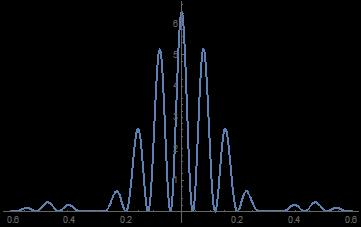Notice that the interference pattern seems like it could fit inside the diffraction pattern shown earlier; we call this the diffraction envelope. In the above example, the distance between the slits was about four times the slit width, and the greater this ratio, the narrower the distance between peaks inside the diffraction envelope. Let me reiterate something: individual particles are detected at the screen. And if we slow down the experiment adequately, we will see individual blips on the screen. None of these blips is, itself, a distribution pattern; rather, the distribution pattern (diffraction in the case of one slit or interference in the case of two) becomes apparent only after measuring lots of lots of blips. Immediately a problem arises in the case of interference. Since individual particles are both emitted by the source and detected at the screen, it certainly seems plausible that individual particles pass through the slits. However, if a particle passes through, say, the left slit, then it should produce a single-slit diffraction pattern, unless the particle somehow knows about the existence of the right slit. Because an interference pattern is actually created, then either: a) The particle, as it passes through the left slit, must instantly know about the existence and size of the right slit, which is located some distance away; or b) It is not the case that the particle passes through the left slit (or the right slit, by similar reasoning). The problem with a) is nonlocality. Special Relativity asserts that information cannot travel faster than the speed of light, which implies that instantaneous transfer of information is impossible. Historically, Special Relativity was proposed by Einstein in 1905, about two decades before the formal creation of quantum wave mechanics. So option a) was summarily dismissed on the grounds that the path of a particle (in this case the transverse momentum component of a particle passing through the left slit) could not possibly be affected by nonlocal information about the right slit located some arbitrary distance away. Consequently, we have been stuck, for nearly a century, with option b). How is it the case that there is no particle that passes the left slit or the right slit, even though a particle was emitted by the source and detected at the screen? Herein lie both the mathematical beauty and the philosophical wackiness of quantum mechanics. Essentially, quantum wave mechanics begins by assuming that the likelihood of finding a particle at a location in space is related to the magnitude of a wave at that point. Because one-dimensional waves are sinusoidal, standing waves have the form eikx. In the case of single-slit diffraction, a wave originating at the slit will spread out radially so that the wave, as measured along the transverse direction, will vary sinusoidally but will also decrease linearly as the radial distance r from the slit. When the distance from the slit is determined almost entirely by the distance between the slit and the screen (i.e., the diffraction angle is small, such as 1°), then the wave in the x direction varies like sin(αx)/αx (also known as sinc(αx)). Then, the likelihood of actually detecting a particle on the screen between two locations is just found by integrating the probability distribution ρ(x) = Ψ*(x) Ψ(x), which looks like the experimentally observed Fraunhofer diffraction, shown previously. Now lets apply this mathematical formalism to the double-slit problem. We now assume that at the location of the plane of the slits we can represent the (particle?) system as a wave Ψ consisting of the superposition of a left-slit wave ΨL and a right-slit wave ΨR so that Ψ(x) = ΨL(x) + ΨR(x). The beauty of this equation is that if we now plot the probability distribution ρ(x) = Ψ*(x) Ψ(x), we get what looks like the experimentally observed interference distribution, shown previously. In other words, if we assume that the system at the location of the slits is not a particle, but a wave that later determines probabilities of detection, then we successfully predict the empirically observed probability distributions. The reason this works, mathematically, is because quantum wave mechanics allows negative probabilities. Look back at the interference distribution and choose some place on it where the probability is zero. If only one slit had been open, the probability of detecting a particle at this point would have been nonzero. So how is it that by adding another slit  by adding another possible path through which a particle could reach point A  that we decrease its likelihood to reach point A? The answer, mathematically, is that by adding waves prior to taking their magnitude, terms that are out of phase can cancel each other, resulting in a sort of negative probability. However, something doesnt make sense. Remember that the wave ΨL(x) is associated with a particle that travels through the left slit and wave ΨR(x) is associated with a particle that travels through the right slit. But what can this possibly mean if we have already assumed that it is not the case that the particle passes through the left slit or the right slit? This is the very heart of the so-called measurement problem. By localizing the particle (or whatever the hell it is) within the two slits, we assume that it is in superposition Ψ(x) = ΨL(x) + ΨR(x). But if we subsequently measure the particle as having come from one of the slits (called a which-way measurement), then we were wrong about its earlier state. And if we were right about its earlier state, then it will forever remain in a superposition. So there is something very weird, and possibly wrong, with option b). So maybe option a) is right and we just have to accept nonlocality. After all, quantum entanglement also seems to require nonlocality, so maybe thats just a fact about the quantum world. Physicist Yakir Aharonov has written a lot on the topic of nonlocality in quantum measurement, such as this. By the way, treating a system with two slits as the superposition of two waves still does not solve the nonlocality problem. After all, consider a single slit of width Δx. This produces a single-slit Fraunhofer diffraction distribution and certainly no one would object to the assertion that every particle detected on the screen actually passed through the slit. (Right??) Of course, if Δx is zero, then theres no problem with Special Relativity. However, no slit has zero width, so lets divide the slit into a left half and a right half. Now, we can associate a wave with each half and treat each half as producing its own Fraunhofer diffraction envelope having double the width. The interference between these two waves then produces an interference pattern that, incredibly enough, is identical to the single-slit Fraunhofer diffraction distribution of the entire slit. In other words, a single-slit diffraction is double-slit interference for side-by-side slits. So we are again left with options a) and b), even for a single slit. It is important to remember that the representation of the system as a complex superposition of mutually exclusive possibilities was (and remains) an assumption. Of course, it is an assumption whose numerical predictions have been empirically tested and confirmed to staggering precision. However, if there is an understanding of the quantum world that yields the same or better predictions while avoiding sloppy philosophical paradoxes, then might that be preferred? Im proposing a different approach. I do not think its original, but frankly after lots of research, I cant find this approach. First, consider a localization experiment of a particle, and lets assume that the particle actually is located somewhere. In the case of a single slit, lets assume that at some time the particle is, in fact, located somewhere in the slit with constant probability; in the case of the double slit, it is located in either slit with equal probability; and so forth. Next, take the Fourier transform of the entire location distribution, then take its magnitude. For some reason, for a square function (corresponding to a single slit), this yields exactly the Fraunhofer diffraction distribution in momentum space. We can then empirically find the relationship between the momentum space and position space by noting that the spread of the distribution in momentum space is inversely proportional to the spread in position space, and their product is on the order of Plancks constant. By the way, I dont yet understand why the magnitude of the Fourier transform of a square function yields the sinc2(αx) distribution so typical of Fraunhofer diffraction, although I suspect it generalizes by starting from a perfect localization down to the Planck length (and the resulting complete lack of knowledge one could have about momentum at this scale). In any event, not only do I find this fact amazing, but I frankly wasnt convinced that p=ℏk was the same momentum as p=mv of a massive object until I noticed that distributions of particles passing through a slit correspond to the magnitude of the Fourier transform of that slit! Finally, assume each particle passing through the localized region can take on transverse momenta according to this distribution, and then integrate this value over the entire localized region. This, I believe, may yield the actual distribution of detected particles. To test my results, I used Mathematica to simulate the situations numerically. In each case I divided the localization region into lots of smaller regions. In one case (called Adding Fields), I added the fields of all the regions first before calculating intensities/probabilities; in the other case (called Adding Probabilities), I calculated intensities/probabilities first and then added the contributions by each region. I made a few assumptions: · The incoming particles had momentum such that the central diffraction envelope spreads at an angle of 1°. · The incoming particles were assumed to come from a point source with effectively no spread in momentum (which, I think, is another way of saying they are assumed to be monochromatic and spatially coherent). For diffraction, I divided the single slit into n regions. In the Adding Fields simulation, I calculated the Fourier transform of each region to find their fields, added the fields of each region, and then plotted the magnitude of this sum for various parameters. In the Adding Probabilities simulation, I calculated the Fourier transform of the entire slit, assumed that each region produces an intensity based on the fields in this total Fourier transform, and then plotted the sum of these intensities for various parameters. Here is a typical example, in which the slit is divided into 20 regions and the screen is a distance of 50 times the slit width: Adding Fields: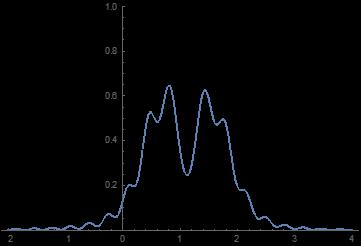Adding Probabilities:A distance of 50 times the slit width is very much in the near field, where we would expect the distribution to be relatively flat (corresponding to the width of the slit), with edge effects that reflect the 1° spread. Only the Adding Probabilities distribution satisfies these expectations. The situation is worse when the slit is divided into 100 regions: Adding Fields:Adding Probabilities:In the far field, such as where the screen is 10,000 times the slit width, both simulations converge to the expected Fraunhofer diffraction distribution. To simulate double-slit interference in the Adding Fields simulation, I simply added another slit of equal width, some distance away, and broken into n regions, and continued the analysis by first adding the fields of each region and then finding the magnitude of their sum. In the Adding Probabilities simulation, I calculated the Fourier transform of both slits together (i.e., the entire localization space), assumed that each region produces an intensity based on the fields in this total Fourier transform, and then plotted the sum of these intensities for various parameters. Here is a typical example, in which the slits are each divided into 100 regions, the slit separation is 10 times the slit width, and the screen is located a distance of 10 times the slit width. The plots are also shown zoomed in to the left peak: Adding Fields: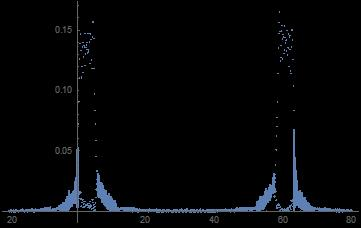Adding Probabilities: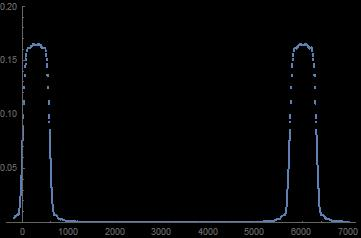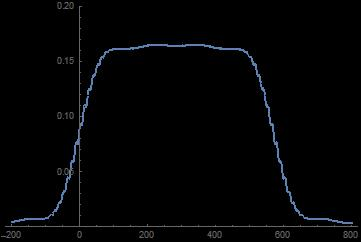Either of these distributions might fit experimental data, however the Adding Probabilities distributions are more plausible. In the far field, starting at around a million times the slit width, both simulations converge to the expected interference pattern. So whats the answer? Is the Adding Probabilities method wrong? For the life of me, I CANNOT FIND THE ANSWER. I have read dozens of papers and scoured the internet, and basically every source says that you add the fields first and then find the probabilities, instead of just doing a Fourier transform on the entire localization space and assuming that each localized particle assumes the resulting momentum distribution. This is also pretty simple, so I seriously doubt Ive discovered something new... which means I must have made a mistake somewhere. There are certainly references (such as this) that say that you add probabilities when the source particles are incoherent, but my analysis seems to apply to any source, including a laser. PLEASE HELP!!Oct 8th 2019, 10:28 AM #2 Senior Member   Join Date: Jun 2016 Location: England Posts: 1,060Not Silly Often when we get an essay posted, it signals a quack, However, I must say that (in my view) your post actually reads quite well! There have been thoughts around your idea, Have a look at the As you yourself note, this treatment of the probability function is inherently non-local. This used to be enough to immediately discard it without further thought, But with entanglement and other QM weirdness's also seeming to require non-locality, people are less prone to dismiss the idea purely on those grounds. I have seen a few articles here and there that suggest people are starting to sniff around the idea again. topsquark and donglebox like this. __________________ ~\o/~Oct 8th 2019, 04:35 PM #3 Junior Member   Join Date: Oct 2019 Posts: 2 Thank you! Thanks Woody... I was already somewhat familiar with deBroglie-Bohm, but I'll take a closer look at it. Also thank you for saying my post isn't silly. I've been struggling with this question for quite a while, and am now taking graduate physics classes (including QM), so I wasn't sure if I was just missing something very simple or if maybe there was more to the question.Oct 8th 2019, 07:46 PM #4 Senior Member   Join Date: Mar 2019 Location: cosmos Posts: 666quantum states vs independent affairs This article from aknight is full of spirit of exploration. He should be a hopeful student. It's not so easy for this semi-tramp to read English and something always can not be shown on the computer screen in his own world. ............................ "Immediately a problem arises in the case of interference. Since individual particles are both emitted by the source and detected at the screen, it certainly seems plausible that individual particles pass through the slits. However, if a particle passes through, say, the left slit, then it should produce a single-slit diffraction pattern, unless the particle somehow knows about the existence of the right slit. Because an interference pattern is actually created, then either: a) The particle, as it passes through the left slit, must instantly know about the existence and size of the right slit, which is located some distance away; or The problem with a) is nonlocality. Special Relativity asserts that information cannot travel faster than the speed of light, which implies that instantaneous transfer of information is impossible. Historically, Special Relativity was proposed by Einstein in 1905, about two decades before the formal creation of quantum wave mechanics. So option a) was summarily dismissed on the grounds that the path of a particle (in this case the transverse momentum component of a particle passing through the left slit) could not possibly be affected by nonlocal information about the right slit located some arbitrary distance away." This semi-tramp's comment is: the authentic theory now can not explain it yet. "Eccentric" hole understand this very clearly, but it's not for pysics students still in school. Actually it's possible...No more talking, sorry. .............................. "...Now lets apply this mathematical formalism to the double-slit problem. We now assume that at the location of the plane of the slits we can represent the (particle?) system as a wave Ψ consisting of the superposition of a left-slit wave ΨL and a right-slit wave ΨR so that Ψ(x) = ΨL(x) + ΨR(x). The beauty of this equation is that if we now plot the probability distribution ρ(x) = Ψ*(x) Ψ(x), we get what looks like the experimentally observed interference distribution, shown previously. In other words, if we assume that the system at the location of the slits is not a particle, but a wave that later determines probabilities of detection, then we successfully predict the empirically observed probability distributions. " This semi-tramp's comment is: yes, this is the answer you should favour now. But your concept about a "particle" should not be that kind of a salted fish. ................................ "...However, something doesnt make sense. Remember that the wave ΨL(x) is associated with a particle that travels through the left slit and wave ΨR(x) is associated with a particle that travels through the right slit. But what can this possibly mean if we have already assumed that it is not the case that the particle passes through the left slit or the right slit?" This semi-tramp's comment is: if you assume "a particle that travels through the left slit ", then the asscociate wave should be denoted as ΨL(x)', rather than ΨL(x); if you assume "...a particle that travels through the right slit", then the asscociate wave should be denoted as ΨR(x)', rather than ΨR(x). The key is below: ΨL(x)' and ΨR(x)' are two different independent affairs, and they can not be added simply; While ΨL(x) and ΨR(x) are two different quantum states of the "whatever the hell it is", and they can superposition linearly. .......................... Another prevailing example of confusing "quantum states" and " independent affairs" is "Schrodinger's cat". .......................... ".. But if we subsequently measure the particle as having come from one of the slits (called a which-way measurement), then we were wrong about its earlier state." This semi-tramp's comment is: no contradiction. It's different affair measured. ........................... "...For the life of me, I CANNOT FIND THE ANSWER." This semi-tramp's comment is: Perhaps wrong. Because history ever developing. More important, you are just a student in school. Most important, you are thoughtful and have the spirit of exploration. A bit more, radical.Oct 9th 2019, 12:51 AM #5 Junior Member   Join Date: Sep 2019 Location: CN GX WZ Posts: 20 Hello, you are a person who can find good problems. There aer answers on these two posts are also helpful in guiding the answers. I hope you can find what you want. My reading ability is unreliable and I haven't gone further in the standard curriculum. You have found that by replacing some function parts, you can get similar images as well. Some tips We may have to distinguish the 1，fitting image, 2，simulate the simulation experiment . 3， explain the theory by computer experiment. Here are three or more computer methods, the differences between them. Reference to the Big Bell Test the difference is how natural effects occur. How to intervene, or depend on your combination. Others tell you not to add probabilistic methods first because of the sequence of data in each coupling interface. Maybe they are thinking about avoiding artificial results.Be careful for problems caused by aggregation of regional data. Different probabilistic methods also bring different details. Different from introducing signals and human choices, human interference should also be avoided in experiments.Tags doubleslit, interference, understandThread ToolsShow Printable VersionEmail this Page Display ModesLinear ModeSwitch to Hybrid ModeSwitch to Threaded ModeSimilar Physics Forum Discussions Thread Thread Starter Forum Replies Last Post kiwiheretic Quantum Physics 10 Dec 22nd 2015 11:38 AM physmo Advanced Optics 2 Jan 14th 2015 07:16 AM ling233 Light and Optics 1 Dec 7th 2014 10:30 AM Awsom Guy Waves and Sound 2 Jun 20th 2010 12:58 AM mola Advanced Optics 1 May 24th 2009 09:34 PM